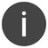# ^DATATYPE

Return the data type

 WTSupported in traditional Synergy on Windows WNSupported in Synergy .NET on Windows USupported on UNIX VSupported on OpenVMS
```^DATATYPE(position)
```

Arguments

position

The ordinal position of the argument whose data type will be returned. (n)

Discussion

^DATATYPE returns the data type of an argument as one of the following values, which are defined by the compiler:

D_TYPE_A = 1 Alpha

D_TYPE_D = 2 Decimal

D_TYPE_ID = 4 Implied-decimal

D_TYPE_I = 8 Integer

D_TYPE_P = 32 Packed

D_TYPE_IP = 36 Implied-packed

D_TYPE_OBJ = 24 Object handle

Use the .IS. operator to determine a specific type of class. (See Object operators for more information about .IS..)In Synergy .NET, ^DATATYPE is not allowed on MISMATCH a arguments; it should only be used on MISMATCH n arguments. (See the ”Parameter definitions” Discussion for more information about using ^DATATYPE with MISMATCH.)

Examples

```subroutine stringval
a_results           ,a
;Implied second parameter
proc
if (^passed(^arg(2))) then
begin
using (^datatype(2)) select
(D_TYPE_A),
a_result = "String is " + ^arg(2)
(D_TYPE_D, D_TYPE_I),
a_result = "Value is " + %string(^argn(2))
(),
a_result = "Value is invalid"
endusing
end
else
a_result = "String or value not passed”
xreturn
endsubroutine
```